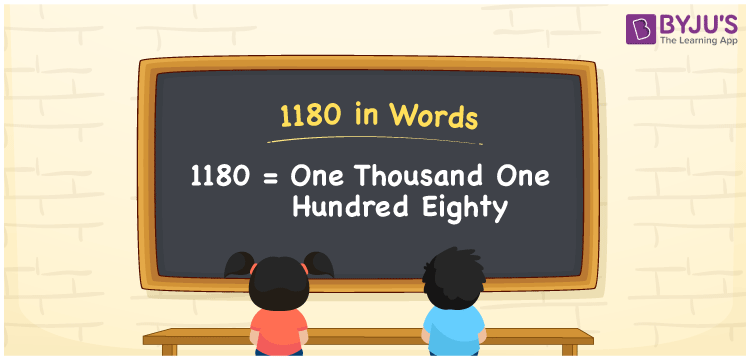# 1180 in Words

One thousand one hundred eighty is represented by the number 1180. When converting numbers to words, the place value chart helps a lot. As a result, you can say that using the place value table for writing 1180 in words is preferable. For example, if you made a transaction of Rs. 1180, you can write “I made a transaction of Rs. One thousand one hundred eighty”. Now let us look at how to spell 1180 in words, as well as the steps that must be followed, with a detailed explanation.

 1180 in Words: One Thousand One Hundred Eighty. One Thousand One Hundred Eighty in Numerical Form: 1180

## 1180 in English Words## How to Write 1180 in Words?

For converting 1180 to words, use a place value chart. Below is a place value table for the number 1180, as well as an expanded form.

 Thousands Hundreds Tens Ones 1 1 8 0

Using a place value chart, the number 1180 is expressed in the form:

= 1 × Thousand + 1 × Hundred + 8 × Ten + 0 × One

= 1 × 1000 + 1 × 100 + 8 × 10

= 1000 + 100 + 80

= 1180

= one thousand one hundred eighty

Hence, 1180 in words is one thousand one hundred eighty.

Following 1179 but just before 1181, the natural number 1180 exists.

1180 in words – One thousand one hundred eighty

Is 1180 an odd number? – No

Is 1180 an even number? – Yes

Is 1180 a perfect square number? – No

Is 1180 a perfect cube number? – No

Is 1180 a prime number? – No

Is 1180 a composite number? – Yes

## Frequently Asked Questions on 1180 in Words

Q1

### Write 1180 in words.

1180 in words is one thousand one hundred eighty.

Q2

### Simplify 1200 – 20, and express in words.

Simplifying 1200 – 20, we get 1180. Hence, 1180 in words is one thousand one hundred eighty.

Q3

### How to write one thousand one hundred eighty in numbers?

One thousand one hundred eighty in numbers is 1180.

Test your Knowledge on 1180 in Words